# 3.1 Functions and function notation  (Page 9/21)

 Page 9 / 21

We will see these toolkit functions, combinations of toolkit functions, their graphs, and their transformations frequently throughout this book. It will be very helpful if we can recognize these toolkit functions and their features quickly by name, formula, graph, and basic table properties. The graphs and sample table values are included with each function shown in [link] .

Toolkit Functions
Name Function Graph
Constant $f\left(x\right)=c,\text{\hspace{0.17em}}$ where $c$ is a constant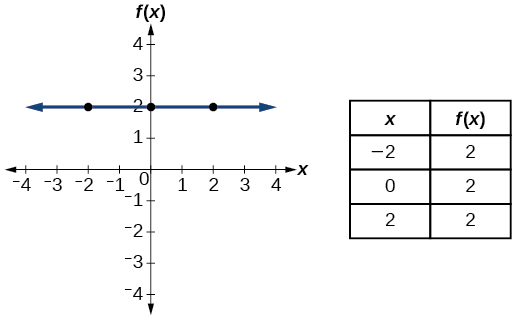Identity $f\left(x\right)=x$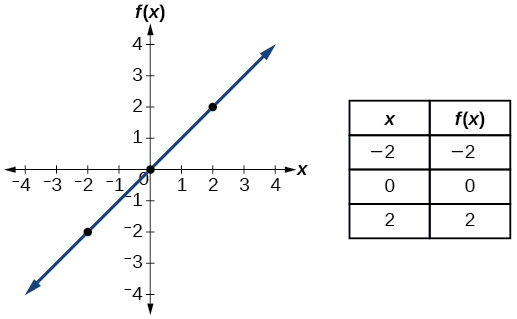Absolute value $f\left(x\right)=|x|$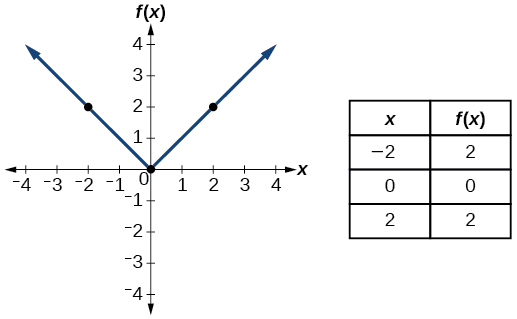Quadratic $f\left(x\right)={x}^{2}$Cubic $f\left(x\right)={x}^{3}$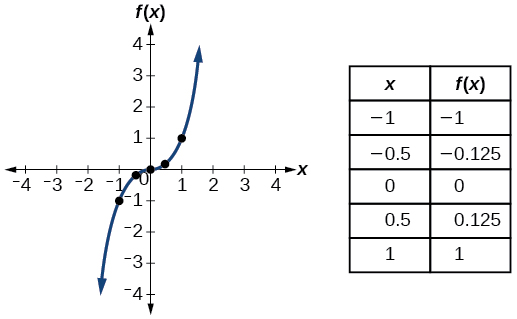Reciprocal $f\left(x\right)=\frac{1}{x}$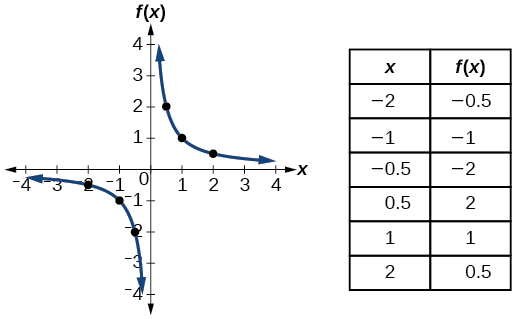Reciprocal squared $f\left(x\right)=\frac{1}{{x}^{2}}$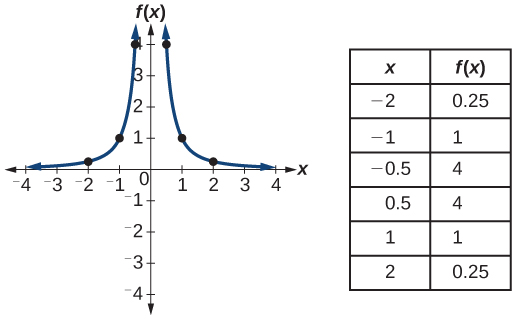Square root $f\left(x\right)=\sqrt{x}$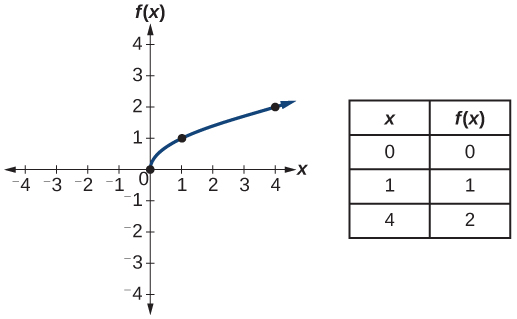Cube root $f\left(x\right)=\sqrt{x}$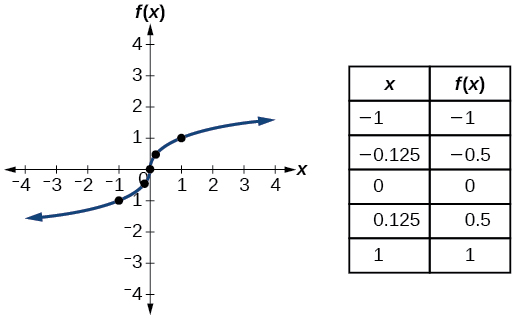Access the following online resources for additional instruction and practice with functions.

## Key equations

 Constant function $f\left(x\right)=c,$ where $\text{\hspace{0.17em}}c\text{\hspace{0.17em}}$ is a constant Identity function $f\left(x\right)=x$ Absolute value function $f\left(x\right)=|x|$ Quadratic function $f\left(x\right)={x}^{2}$ Cubic function $f\left(x\right)={x}^{3}$ Reciprocal function $f\left(x\right)=\frac{1}{x}$ Reciprocal squared function $f\left(x\right)=\frac{1}{{x}^{2}}$ Square root function $f\left(x\right)=\sqrt{x}$ Cube root function $f\left(x\right)=\sqrt{x}$

## Key concepts

• A relation is a set of ordered pairs. A function is a specific type of relation in which each domain value, or input, leads to exactly one range value, or output. See [link] and [link] .
• Function notation is a shorthand method for relating the input to the output in the form $\text{\hspace{0.17em}}y=f\left(x\right).\text{\hspace{0.17em}}$ See [link] and [link] .
• In tabular form, a function can be represented by rows or columns that relate to input and output values. See [link] .
• To evaluate a function, we determine an output value for a corresponding input value. Algebraic forms of a function can be evaluated by replacing the input variable with a given value. See [link] and [link] .
• To solve for a specific function value, we determine the input values that yield the specific output value. See [link] .
• An algebraic form of a function can be written from an equation. See [link] and [link] .
• Input and output values of a function can be identified from a table. See [link] .
• Relating input values to output values on a graph is another way to evaluate a function. See [link] .
• A function is one-to-one if each output value corresponds to only one input value. See [link] .
• A graph represents a function if any vertical line drawn on the graph intersects the graph at no more than one point. See [link] .
• The graph of a one-to-one function passes the horizontal line test. See [link] .

## Verbal

What is the difference between a relation and a function?

A relation is a set of ordered pairs. A function is a special kind of relation in which no two ordered pairs have the same first coordinate.

What is the difference between the input and the output of a function?

Why does the vertical line test tell us whether the graph of a relation represents a function?

When a vertical line intersects the graph of a relation more than once, that indicates that for that input there is more than one output. At any particular input value, there can be only one output if the relation is to be a function.

How can you determine if a relation is a one-to-one function?

what are you up to?
nothing up todat yet
Miranda
hi
jai
hello
jai
Miranda Drice
jai
aap konsi country se ho
jai
which language is that
Miranda
I am living in india
jai
good
Miranda
what is the formula for calculating algebraic
I think the formula for calculating algebraic is the statement of the equality of two expression stimulate by a set of addition, multiplication, soustraction, division, raising to a power and extraction of Root. U believe by having those in the equation you will be in measure to calculate it
Miranda
state and prove Cayley hamilton therom
hello
Propessor
hi
Miranda
the Cayley hamilton Theorem state if A is a square matrix and if f(x) is its characterics polynomial then f(x)=0 in another ways evey square matrix is a root of its chatacteristics polynomial.
Miranda
hi
jai
hi Miranda
jai
thanks
Propessor
welcome
jai
What is algebra
algebra is a branch of the mathematics to calculate expressions follow.
Miranda
Miranda Drice would you mind teaching me mathematics? I think you are really good at math. I'm not good at it. In fact I hate it. 😅😅😅
Jeffrey
lolll who told you I'm good at it
Miranda
something seems to wispher me to my ear that u are good at it. lol
Jeffrey
lolllll if you say so
Miranda
but seriously, Im really bad at math. And I hate it. But you see, I downloaded this app two months ago hoping to master it.
Jeffrey
which grade are you in though
Miranda
oh woww I understand
Miranda
Jeffrey
Jeffrey
Miranda
how come you finished in college and you don't like math though
Miranda
gotta practice, holmie
Steve
if you never use it you won't be able to appreciate it
Steve
I don't know why. But Im trying to like it.
Jeffrey
yes steve. you're right
Jeffrey
so you better
Miranda
what is the solution of the given equation?
which equation
Miranda
I dont know. lol
Jeffrey
Miranda
Jeffrey
answer and questions in exercise 11.2 sums
how do u calculate inequality of irrational number?
Alaba
give me an example
Chris
and I will walk you through it
Chris
cos (-z)= cos z .
cos(- z)=cos z
Mustafa
what is a algebra
(x+x)3=?
6x
Obed
what is the identity of 1-cos²5x equal to?
__john __05
Kishu
Hi
Abdel
hi
Ye
hi
Nokwanda
C'est comment
Abdel
Hi
Amanda
hello
SORIE
Hiiii
Chinni
hello
Ranjay
hi
ANSHU
hiiii
Chinni
h r u friends
Chinni
yes
Hassan
so is their any Genius in mathematics here let chat guys and get to know each other's
SORIE
I speak French
Abdel
okay no problem since we gather here and get to know each other
SORIE
hi im stupid at math and just wanna join here
Yaona
lol nahhh none of us here are stupid it's just that we have Fast, Medium, and slow learner bro but we all going to work things out together
SORIE
it's 12
what is the function of sine with respect of cosine , graphically
tangent bruh
Steve
cosx.cos2x.cos4x.cos8x
sinx sin2x is linearly dependent
what is a reciprocal
The reciprocal of a number is 1 divided by a number. eg the reciprocal of 10 is 1/10 which is 0.1
Shemmy
Reciprocal is a pair of numbers that, when multiplied together, equal to 1. Example; the reciprocal of 3 is ⅓, because 3 multiplied by ⅓ is equal to 1
Jeza
each term in a sequence below is five times the previous term what is the eighth term in the sequence
I don't understand how radicals works pls
How look for the general solution of a trig functionByByBy Qqq QqqBy DanielrosenbergerByBy Jonathan LongBy Maureen MillerBy OpenStaxBy OpenStaxBy OpenStaxBy Dionne MahaffeyBy Alec Moffit